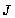NATIONAL ACADEMY OF SCIENCES OF UKRAINE PIDSTRYHACH INSTITUTE OF APPLIED PROBLEMS OF MECHANICS AND MATHEMATICS

Scientific Journal
"MATHEMATICAL METHODS and PHYICOMECHANICAL FILDS"

Year 2003, Vol.46
 Vol. 46, No.4, L'viv 2003
 7 CONTENTS Antonova T. M. Convergence of branched continued fractions with complex ele�ments and their even parts Hladun V. R. Conditions of convergence and stability of branched continued frac�tions with negative partial numerators 16 Baran O. Ye. 27 Estimations of speed of convergence of interpolation process based on branched continued fraction with unequal variables Manzij O. S. 33 Estimation of speed of convergence of Nörlund continued fraction Dmytryshyn R. I. 39 On some regions of convergence of multidimensional-fraction Hoyenko N. P. 44 Application of multidimensional analogue of Worpitzky theorem to investigation of convergence of hypergeometric Lauricella functions expansion in branched continued fractions Nedashkovskyy M. O. 50 Convergence criteria of matrix branched continued fractions Pagirya �. �. 57 On function approximation effectiveness of some types of interpolation continued fractions Chyp M. M. 65 Method of moments for function representation by series and integral Myl�o O. Ya., Pipa H. M., Storozh O. G. 73 On Weyl function and extremal extensions of semi-smooth restriction of positively defined operator Kyrchei I. I. 81 Analogue of adjoint matrix over skew field with involution Symotyuk M. M., Medvid O. M. 92 Problem with integral conditions for linear partial differential equations with constant coefficients Malitska H. P. On stabilization of Poisson�s integral for ultraparabolic equations 102 Jabłoński W., Pękala A. Stability of generalized mean value type functional equation 110 Prokopovych I. B. 114 On differentiation with respect to bound tensor argument Kutniv M. V. 120 Modified three-point difference schemes of high accuracy order for the second-order monotone ordinary differential equations Bomba A. Ya., Pr�gorn�tsky D. O. 130 Approximation of solutions to one class of inverse boundary-value problems on conformal mappings in multiply connected areas with control potential Slivka A. I. 138 Properties of solution to problem on rectangular membrane vibration under random initial conditions Rogowski B. Torsion of fiber-reinforced composite with cylindrical interface crack 145 Yevtushenko A. A., Ivanyk E. G., Rożniakowski K., Dobryansky I. M., Wypych W. Model of thermal fracture of concrete during laser irradiation 154 Kindratskyy B. I., Sulym G. T. Structural-parametric synthesis of metal work of linear motion module 162 Alphabetical index for 2003 (vol. 46) 170